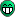# Fitting multi-peak spectum with "shortcuts"

I’ve started to search for a solution of the following problem.

Assume you have a (one-dimensional) “spectrum” (in form of a TH1F, TH1D, TGraph -> suit yourself).
That spectrum contains several (of the order of 1 to 10) “bell-shaped curves” -> I define my own “shape” in C++ (e.g. a “distorted gaussian” with two additional distortion parameters, “tau_1” and “tau_2”, and an “eta” parameter, which says when to switch from the first distortion to the second one) -> something like:
double Peak(double x, double area, double mean, double sigma, double tau_1, double eta, double tau_2);
double Peak(double *x, double *p) { return Peak(x, p, p, p, p, p, p); }
TF1 *FPeak = new TF1(“FPeak”, Peak, 0, 1024, 6); // 1 peak = 6 parameters

Thus, in the most general case, each “peak” needs 6 parameters.

In “standard” ROOT, in order to fit my “spectrum” with “n” peaks, I always need to create a “spectrum specific” C++ function and then a TF1 out of it (i.e. summing up “n” peaks, which, in the most general case, will need “n * 6” parameters).

Now, I’ve met the problem that, for some reasons, the “mean” of one peak should be the same as the "mean " of another one, and the “sigmas” of several peaks should be the same, and some “areas” should be the same, …
The number of possible “combinations” of these “shortcuts” is much too high to create “case specific” C++ functions and TF1s for each one of them.

So, I started to think that I need a solution which first defines a list of “available” parameters (unique “areas”, “means”, “sigmas”, …) and then somehow “adds” peaks, specifying which of “parameters” should be used for which peak.
The resultant (TF1) “function” will be used to fit the “spectrum”, of course.
(It should also be possible to set parameters as “fixed” and/or “limited”.)

Any ideas how to get it rolling?

Well, I have no practice with anything that’s related to any “Roo” (comes from another fairy-tale, you know), but now, maybe becoming familiar with “RooFit” would help?